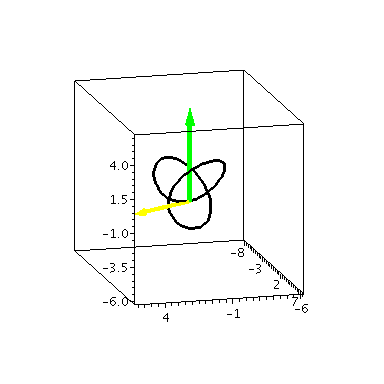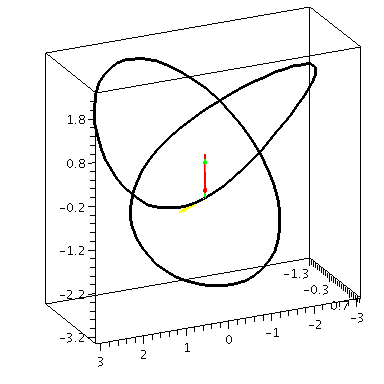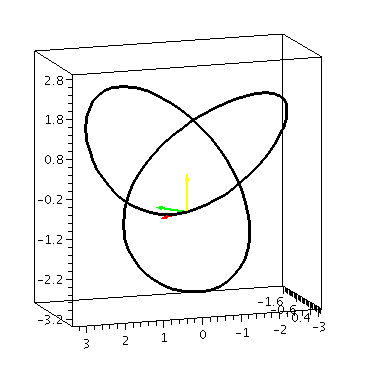# Examples from Section 13.3

The following examples use the trefoil knot for the space curve. Our scaling and choice of coordinates gives:
x = sin(t) + 2 sin(2t)
y = sin(3t)
z = cos(t) - 2 cos(2t)
The first animation below shows three vectors: The red vector is position, the velocity vector is yellow and the acceleration is green. The lengths correspond to the magnitude of each:The next animation shows the vectors scaled back for better viewing. Again, red is position, yellow is velocity, acceleration is green.The next animation shows the Frenet frame (a.k.a. TNB frame) for the trefoil knot- The unit tangent is red, the unit normal is yellow, and the binormal vector is green.